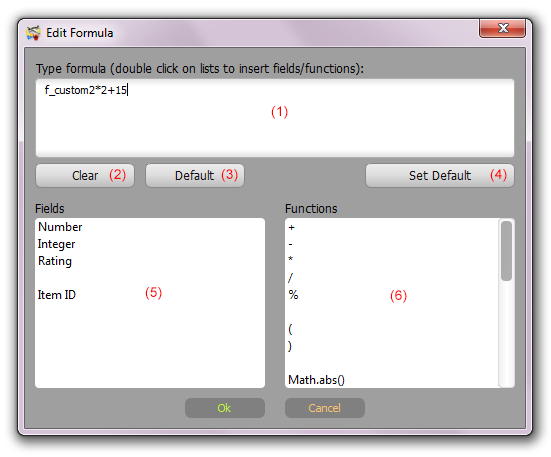Using formulas

Multi Collector allows the use of any kind of standardized ECMAScript (JavaScript/Java). They look like standard formulas. Any fields in the database can be accessed using 'f_' prefix.

item_id is used to get a unique item number. This can be used as an auto-increment identifier.

Examples:
```f_price*3+20 (f_price*f_quantity+25)/10 item_id Math.abs(f_id1-f_id2) Math.min(f_id1, f_id2*10) Math.sqrt(f_radius*Math.PI)```

A lot of Java/JavaScript examples can be found on the Internet.Formula Editor contains a Fields (5) and Functions (6) list with available database fields and the most popular functions. Double-click item in the list to auto-insert it to the formula.

The customer can set a personal formula, or use a default formula. If you leave blank a formula field (1) for your item, Multi Collector will use the default formula.

Buttons:

'Clear' (2) - Clear the field formula (use default formula).

'Default' (3) - Show the default formula for this field.

'Set Default' (4) - Set current formula as a default for all items.